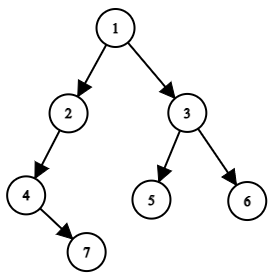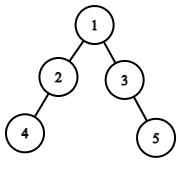# Time To Burn Tree | CodeStudio Solution

## Time To Burn Tree | CodeStudio Solution

Problem Statement
Suggest Edit

#### It is given that it takes 1 minute for the fire to travel from the burning node to its adjacent node and burn down the adjacent node.

##### For Example :
``````For the given binary tree: [1, 2, 3, -1, -1, 4, 5, -1, -1, -1, -1]
Start Node: 3

1
/ \
2   3
/ \
4   5

Output: 2

Explanation :
In the zeroth minute, Node 3 will start to burn.
After one minute, Nodes (1, 4, 5) that are adjacent to 3 will burn completely.
After two minutes, the only remaining Node 2 will be burnt and there will be no nodes remaining in the binary tree.
So, the whole tree will burn in 2 minutes.
``````
##### Input Format :
``````The first line contains elements of the tree in the level order form. The line consists of values of nodes separated by a single space. In case a node is null, we take -1 in its place.

The second line of input contains the value of the start node.
````````````For example, the input for the tree depicted in the above image would be :

1
2 3
4 -1 5 6
-1 7 -1 -1 -1 -1
-1 -1
``````
##### Explanation :
``````Level 1 :
The root node of the tree is 1

Level 2 :
Left child of 1 = 2
Right child of 1 = 3

Level 3 :
Left child of 2 = 4
Right child of 2 = null (-1)
Left child of 3 = 5
Right child of 3 = 6

Level 4 :
Left child of 4 = null (-1)
Right child of 4 = 7
Left child of 5 = null (-1)
Right child of 5 = null (-1)
Left child of 6 = null (-1)
Right child of 6 = null (-1)

Level 5 :
Left child of 7 = null (-1)
Right child of 7 = null (-1)
``````
##### Note :
``````The above format was just to provide clarity on how the input is formed for a given tree.
The sequence will be put together in a single line separated by a single space. Hence, for the above-depicted tree, the input will be given as:

1 2 3 4 -1 5 6 -1 7 -1 -1 -1 -1 -1 -1
``````
##### Output Format
``````Print a single integer denoting the time in minutes that will be taken to burn the whole tree.
``````
##### Note:
``````You do not need to print anything, it has already been taken care of. Just implement the given function.
``````
##### Constraints :
``````1 <= N <= 10^5
1 <= Value of Tree Node <= 10^9
1 <= Value of Start Node <= 10^9

Time limit: 1 sec
``````
##### Sample Input 1 :
``````1 2 3 4 -1 -1 5 -1 -1 -1 -1
2
``````
##### Sample Output 1 :
``````3
``````
##### Explanation Of Sample Input 1 :
``````The given binary tree will look as -
````````````The Start node is 2.
In the zeroth minute, Node 2 will start to burn.
After one minute, Nodes 1 and 4 that are adjacent to 2 will burn completely.
After two minutes, Node 3 that is adjacent to node 1 will burn completely.
After three minutes, the only remaining Node 5 will be burnt and there will be no nodes remaining in the binary tree i.e the whole tree will burn in 3 minutes.
``````
##### Sample Input 2 :
``````3 1 2 5 6 -1 -1 -1 -1 -1 -1
3
``````
##### Sample Output 2 :
``2``
`/************************************************************    Following is the Binary Tree node structure    class BinaryTreeNode     {        public :         T data;        BinaryTreeNode<T> *left;        BinaryTreeNode<T> *right;        BinaryTreeNode(T data)         {            this -> data = data;            left = NULL;            right = NULL;        }    };************************************************************/    #include<bits/stdc++.h>    int ans=INT_MIN;    BinaryTreeNode<int>* parent=NULL;    unordered_map<int,bool>visited;    unordered_map<int,BinaryTreeNode<int>*>m;    void helper(BinaryTreeNode<int>* root,int k){        if(!root)return;        visited[root->data]=true;        ans=max(ans,k+1);        if(root->left && !visited[root->left->data]) helper(root->left,k+1);        if(root->right && !visited[root->right->data])helper(root->right,k+1);        auto it=m.find(root->data);        // cout<<root->val;        if(it!=m.end() && !visited[it->second->data])helper(it->second,k+1);    }        void inorder(BinaryTreeNode<int>* root,int start){        if(!root)return;        // cout<<root->val;        if(root->data==start)parent=root;        if(root->left)m[root->left->data]=root;        if(root->right)m[root->right->data]=root;        inorder(root->left,start);        inorder(root->right,start);    }     int timeToBurnTree(BinaryTreeNode<int>* root, int start){   int k=0;    inorder(root,start);    helper(parent,k);    return ans-1;    // Write your code here}`